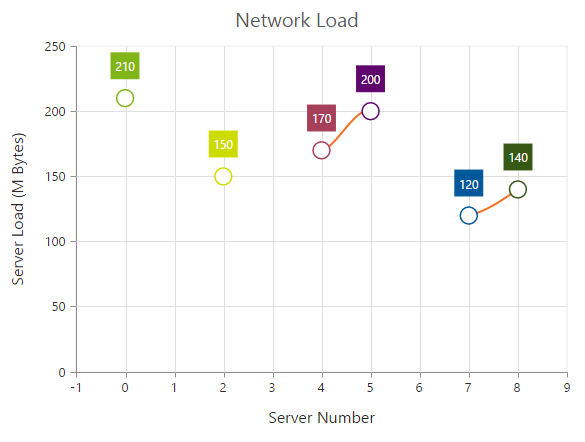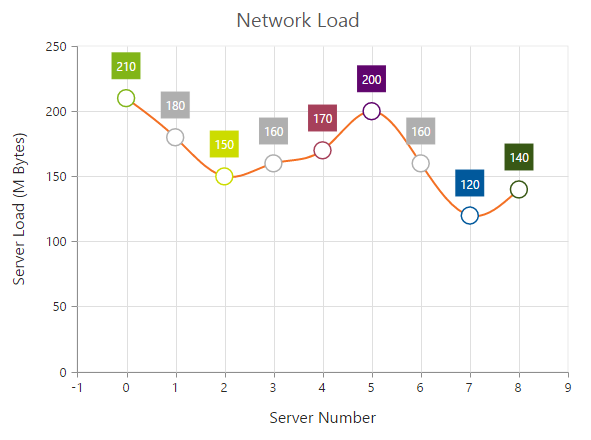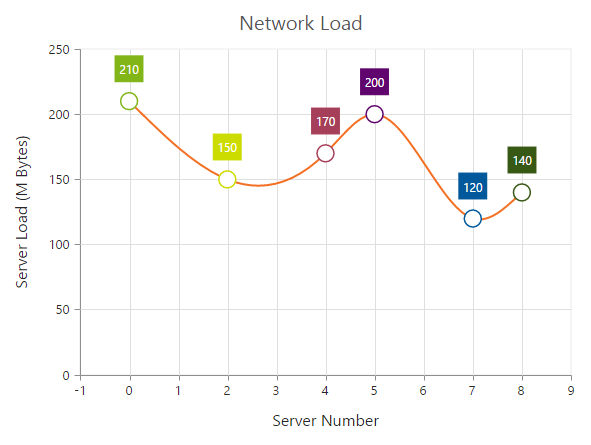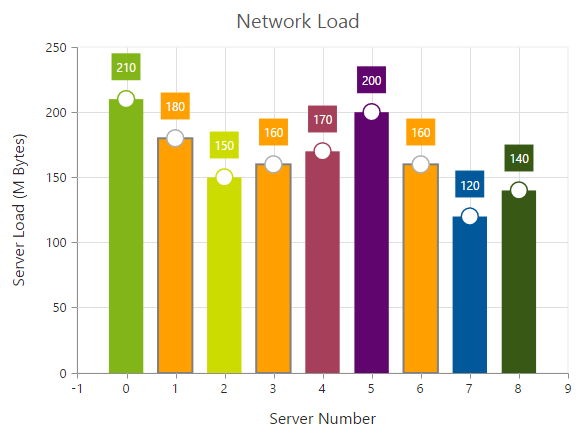# Empty Points

The Data points that uses the null or undefined as value are considered as empty points. Empty data points are ignored and not plotted in the Chart. When the data is provided by using the Points property, you can set the IsEmpty to true to specify that the particular point is an empty point.

• html
• ``````<ej:Chart ID="Chart1" runat="server">
<Series>
<ej:Series>
//Using empty points
<Points>
<ej:Points X="0" Y="210" />
<ej:Points X="1" Y="60" IsEmpty="true"/>
<ej:Points X="2" Y="150" />
<ej:Points X="3" Y="180" IsEmpty="true"/>
<ej:Points X="4" Y="170" />
<ej:Points X="5" Y="200"/>
<ej:Points X="6" Y="140" IsEmpty="true"/>
<ej:Points X="7" Y="120" />
<ej:Points X="8" Y="140" />
</Points>
</ej:Series>
</Series>
</ej:Chart>``````## EmptyPointSettings

You can customize the empty points visibility and change its DisplayMode (Gap, Zero and Average) using EmptyPointSettings option.

• html
• ``````<ej:Chart ID="Chart1" runat="server">
<Series>
<ej:Series>
// visible the EmptyPointSettings width DisplayMode as Average
<EmptyPointSettings Visible="true" DisplayMode="Average"/>
</ej:Series>
</Series>
</ej:Chart>``````If the Visible property of EmptyPointSettings is false, then the empty points has been dropped and chart will be rendered without empty points.## Customizing Styles

Empty points color and border can be customized using Style property of EmptyPointSettings.

• html
• ``````<ej:Chart ID="Chart1" runat="server">
<Series>
<ej:Series>
<EmptyPointSettings Visible="true">
<Style Color="Pink">
<Border Color="Gray" Width="2"/>
</Style>
</EmptyPointSettings>
</ej:Series>
</Series>
</ej:Chart>``````